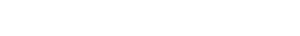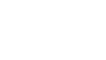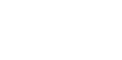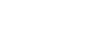# Mastering Basic Differentiation Rules | Cambridge IGCSE Mathematics[Please watch the video attached at the end of this blog for a visual explanation on Mastering Basic Differentiation Rules]

Differentiation is a key lesson that you might have to learn throughout your Mathematics life, especially if you wish to pursue further studies in the field of Mathematics.

### What is Differentiation?

Differentiation is the rate at which one quantity changes with respect to another.

Differentiation is the ratio of a slight change in one quantity to a little change in another that depends on the first quantity. Differentiation is the process of determining the maximum or lowest value of a function, the speed and acceleration of moving objects, and the tangent of a curve. If y = f(x) and f(x) is differentiable, then f'(x) or dy/dx is used to indicate the differentiation. So generally if there is a formula  where y equals something (y = ?), then we call the differentiated value “derivative”.

dy/dx is the name given to the differentiated version of the formula.

### General rule regarding differentiation

The general rule to remember when solving differentiation questions is this:

if y = kxn, then dy/dx (the derivative) can be calculated by multiplying this power to the front. This means we will multiply the value of k times n. Then we consider xn, and we subtract 1 from the given power, i.e. n-1.### Example 1:

We can first of all break this into three parts and handle them one by one.

1. Differentiating 3x2When differentiating 3x2, remember the 2 (n value) multiplies to the front.Therefore 2 ✕ 3 =6And then when it comes to the powers, we subtract 1 from 2 (as we are supposed to consider n-1), so that becomes x1.We have 6x as the differentiation of 3x2.2. Differentiating – 5x.

In – 5x, we can consider x as x1. We can insert an imaginary 1 there, so we multiply that 1 to the front.

1 ✕ -5 = – 5

Then we can consider the powers, we subtract 1 from 1, as n = 1 in this case. Then this will result in – 5x0.

Remember that x0 is the same as saying 1. Therefore we would be left with – 5 when we differentiate – 5x.3.Differentiating + 3

Finally, another thing to always remember is that when differentiating a constant, the constant will always disappear and become zero, so it does not get considered in the final expression.

Hence in this case, the differentiated value of + 3 will be 0.

After getting all the values, we simply put them together!### Example 2:

Find the gradient of the curve y = x – x-1 at the point (2 , 3/2)

dy/dx actually gives us the gradient formula, which allows us to calculate the gradient at any particular point on the line or the curve given.

So here, if the curve is y = x – x-1, we can first differentiate it to find the gradient formula.

We can divide the equation into two parts.

1. Differentiating xWhen we differentiate x, we consider x to have the power 1, i.e. n = 1. Therefore, we bring 1 front and multiply it with x. Then we check the powers which would be n-1. This will result in x0, the resulting answer being 1.
2. Differentiating – x-1

In differentiating – x-1 it will become x-2 when following the same rules as above.Once we have done that, we can actually remove this negative power in using the rules we learnt under the lesson Exponents and Surds. In order to make the power given positive, we make that value the denominator, as 1/x2.This leaves us with 1+1/x2 as the derivative.Since we wish to find out what the gradient is, we must take the x value and substitute that value into the gradient formula.

Then the equation will become 1 + ¼, which is 5/4.

The gradient at that particular point is therefore, 5/4.### Example 3:

There may also be questions where you are asked to find the equation of the tangent to a particular curve and this involves a few steps.

Find the equation of the tangent to the curve 𝑦 = 𝑥3 − 3𝑥2 + 2 at point P where 𝑥 = 3

The first step is to find the gradient at that particular point

We have been given the equation for the curve. We start by differentiating the curve equation. This will result in 3x2  – 6x. When we substitute the x value as 3 (due to the question given), we can find the gradient quite easily. In this case, it will be 9.We have found the gradient which is 9, which will be crucial in finding the equation of the tangent. We have the x value, but we also need the y value, so an additional step we must do is we take the initial equation given to us, and we substitute the value of x which is 3, and we find out the value of y.Once we obtain the information that the value of y or the coordinates of point P are 3,2, we can substitute the value of y. We know that any line has an equation y = mx + c, so for this particular line, we can substitute in the y value as 2, x value as 3 and m value as 9 as per the calculations. This leads to the answer of c being – 25.By substituting all these in, we can then find out that the equation of the tangent is therefore y = 9x – 25.Mastering Basic Differentiation Rules

Read through the rules carefully and ensure you understand them carefully. Always make sure to practise differentiation questions as students tend to confuse this area with many other areas. You can find some questions in this quiz to check where you stand!

If you are struggling with IGCSE revision or the Mathematics subject in particular, you can reach out to us at Tutopiya to join revision sessions or find yourself the right tutor for you.

Watch the video below for a visual explanation of the lesson on mastering basic differentiation rules.